# what inscribed angles and central angles are not very clearly defined. What do they mean?

• Module 2 Week 2 Day 6 Challenge Explanation Part 4

This video is pretty nice but what inscribed angles and central angles are not very clearly defined. What do they mean?

• Dear Xiao Ma,

Thanks for the great question! Po describes these at 5:30 in the challenge explanation video, with visual aids to help. Inscribed angles are those coming from a point on the circle itself, with lines extending to two other points on the circle. Central angles are those which come from the center of the circle, with lines extending to two other points on the circle.

More technically, the inscribed angles are those made on the inside of the circle by two secant lines, and central angles are the angles made when two radii intersect.

I hope that clears things up!

Happy Learning,
The Daily Challenge Team

• How does two inscribed angles equal one central angle?

• Hi Xiao Ma,

This is a very important theorem, so this is a good question to ask! Let me know if there's any part of this explanation you don't get, it's a good test of your current understanding of angles!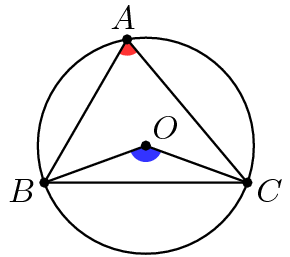We want to prove that the angle \(\angle BOC\), marked in blue, is twice the measure of the angle \(\angle BAC\), marked in red. How can we do this?

The key idea is that we need to use a key piece of information: \(O\) is the center of the circle! That means that \(A\), \(B\), and \(C\) are each the same distance away from \(O\). To make the most use of this, we should probably draw the missing radius! This will split the red angle into two parts, and maybe that will be easier to work with.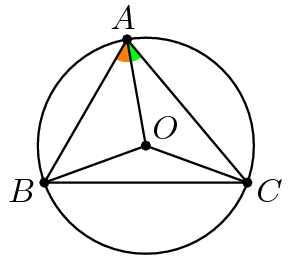I separated the red angle into two different angles, so \(\angle BAC = \angle BAO + \angle OAC\). I marked them in orange and green. Can you find another angle with the same measure as the orange angle? What about the green angle?

We can use the fact that \(AO\), \(BO\), and \(CO\) are all radii of the circle! That means they all have the same length. That means \(\triangle OBA\) and \(\triangle OAC\) are both isosceles. So, \(\angle OAB = \angle OBA\), and \(\angle OAC = \angle OCA\).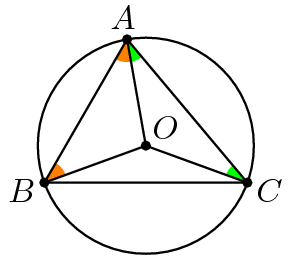Hmm, but now what? How do we get to \(\angle BOC\), the angle that'we care about? Here are two ways to finish.

Method 1

Let's try assigning some variables to the angle measures we have so far.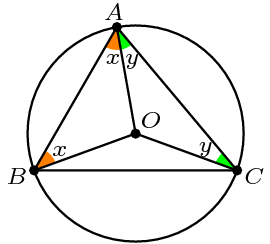Can we write the measure of \(\angle BOA\) in terms of \(x\)? Well, the three angles \(\angle BOA\), \(x\), and \(x\) make a triangle, so they have to add to \(180\). That means that \(\angle BOA = 180^\circ - 2x\). Similarly, \(\angle COA = 180^\circ - 2y\).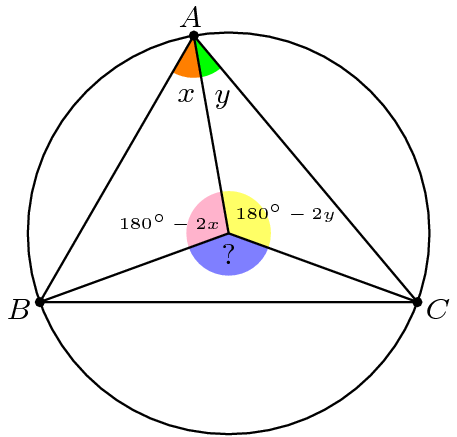We're almost there! How do we find that missing blue angle? As a hint, what do we know about the pink, yellow and blue angles? Well, they make up a "full angle", so they have to add up to \(360^\circ\)! The measure for the blue angle that makes this true would be \(2x+2y\).

So the red angle (from before) is \(x+y\), and the blue angle is \(2x+2y\). So indeed, the blue angle is twice the measure of the red angle!

Method 2

I won't go through all the details, so see if you can figure out the rest by yourself. First, we set variables \(\angle OAB = x\) and \(\angle OAC = y\), as before. But let's also let \(\angle OBC = \angle OCB = z\). Can you write the measure of \(\angle BOC\) in terms of \(z\)? Next, can you write \(z\) in terms of \(x\) and \(y\)? (Hint: What is \(x+x+y+y+z+z\)?)

Anyways, that's the explanation for why the central angle is double the measure of a corresponding inscribed angle. Let me know if you want more detail for any of these steps. Happy learning!